IT数码 购物 网址 头条 软件 日历 阅读 图书馆
 TxT小说阅读器 ↓语音阅读,小说下载,古典文学↓ 图片批量下载器 ↓批量下载图片,美女图库↓ 图片自动播放器 ↓图片自动播放器↓ 一键清除垃圾 ↓轻轻一点,清除系统垃圾↓
 开发: C++知识库 Java知识库 JavaScript Python PHP知识库 人工智能 区块链 大数据 移动开发 嵌入式 开发工具 数据结构与算法 开发测试 游戏开发 网络协议 系统运维 教程: HTML教程 CSS教程 JavaScript教程 Go语言教程 JQuery教程 VUE教程 VUE3教程 Bootstrap教程 SQL数据库教程 C语言教程 C++教程 Java教程 Python教程 Python3教程 C#教程 数码: 电脑 笔记本 显卡 显示器 固态硬盘 硬盘 耳机 手机 iphone vivo oppo 小米 华为 单反 装机 图拉丁
 -> 人工智能 -> pytorch之深入理解collate_fn -> 正文阅读

### 作用

collate_fn：即用于collate的function，用于整理数据的函数。

1. 这两个你如何定义;
2. 从装载器`dataloader`中取数据后做什么处理;
3. 模型的`forward()`中如何处理。

emmm…，小小总结，collate_fn笼统的说就是用于整理数据，通常我们不需要使用，其应用的情形是：各个数据长度不一样的情况，比如第一张图片大小是28*28,第二张是50*50，这样的话就如果不自己写collate_fn，而使用默认的，就会报错。

### 基础

#### dataset

``````import torch.utils.data as Data
class mydataset(Data.Dataset):
def __init__(self,train_inputs,train_targets):#必须有
super(mydataset,self).__init__()
self.inputs=train_inputs
self.targets=train_targets

def __getitem__(self, index):#必须重写
return self.inputs[index],self.targets[index]

def __len__(self):#必须重写
return len(self.targets)
``````
``````#构造训练数据
datax=torch.randn(4,3)#构造4个输入
datay=torch.empty(4).random_(2)#构造4个标签
``````
``````#制作dataset
dataset=mydataset(datax,datay)
``````

``````len(dataset)#调用了你上面定义的def __len__()那个函数
#4
``````
``````dataset#调用了你上面定义的def __getitem__()那个函数
#(tensor([-1.1426, -1.3239,  1.8372]), tensor(0.))
``````

``````    def __getitem__(self, index):
return self.inputs[index]#不输出标签
``````

``````dataset#此时当然变化。
#tensor([-1.1426, -1.3239,  1.8372])
``````

`torch.utils.data.DataLoader`

``````dataloader=Data.DataLoader(dataset,batch_size=2)
``````

4个数据，`batch_size=2`,所以一共有2个`batch`
`collate_fn`如果你不指定，会调用pytorch内部的，也就是说这个函数是一定会调用的，而且调用这个函数时pytorch会往这个函数里面传入一个参数`batch`

``````def my_collate(batch):
return xxx
``````

``````print(datax)
print(datay)
batch=[dataset,dataset]#所以才说和你dataset中get_item的定义有关。
print(batch)
``````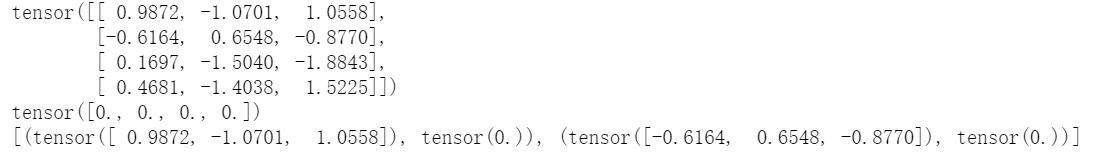``````it=iter(dataloader)
nex=next(it)#我们展示第一个batch经过collate_fn之后的输出结果
print(nex)
``````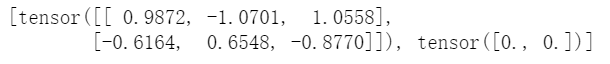``````# a simple custom collate function, just to show the idea
# `batch` is a list of tuple where first element is input tensor and the second element is corresponding label
def my_collate(batch):
inputs=[data.tolist() for data in batch]
target = torch.tensor([data for data in batch])
return [data, target]
``````
``````dataloader=Data.DataLoader(dataset,batch_size=2,collate_fn=my_collate)
``````
``````print(datax)
print(datay)
``````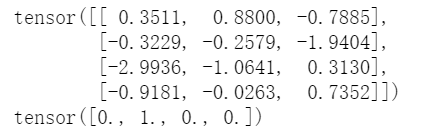``````it=iter(dataloader)
nex=next(it)
print(nex)
``````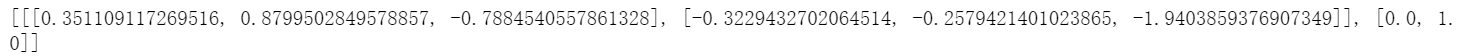``````def my_collate(batch):
inputs=[data.tolist() for data in batch]
inputs=torch.tensor(inputs)
target =[data.tolist() for data in batch]
target=torch.tensor(target)
return [inputs, target]

``````
``````dataloader=Data.DataLoader(dataset,batch_size=2,collate_fn=my_collate)
``````
``````it=iter(dataloader)
nex=next(it)
print(nex)
``````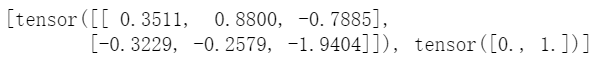### 应用情形

``````a=[[1,2],[3,4,5],,[3,4,9]]
b=[1,0,0,1]
dataset=mydataset(a,b)
nex=next(it)
nex
``````上一篇文章      下一篇文章      查看所有文章
 加:2021-09-26 10:10:08  更:2021-09-26 10:12:20
 开发: C++知识库 Java知识库 JavaScript Python PHP知识库 人工智能 区块链 大数据 移动开发 嵌入式 开发工具 数据结构与算法 开发测试 游戏开发 网络协议 系统运维 教程: HTML教程 CSS教程 JavaScript教程 Go语言教程 JQuery教程 VUE教程 VUE3教程 Bootstrap教程 SQL数据库教程 C语言教程 C++教程 Java教程 Python教程 Python3教程 C#教程 数码: 电脑 笔记本 显卡 显示器 固态硬盘 硬盘 耳机 手机 iphone vivo oppo 小米 华为 单反 装机 图拉丁
 360图书馆 购物 三丰科技 阅读网 日历 万年历 2021年10日历 -2021/10/28 13:13:32-
 图片自动播放器 ↓图片自动播放器↓ TxT小说阅读器 ↓语音阅读,小说下载,古典文学↓ 一键清除垃圾 ↓轻轻一点,清除系统垃圾↓ 图片批量下载器 ↓批量下载图片,美女图库↓
 网站联系: qq:121756557 email:121756557@qq.com  IT数码